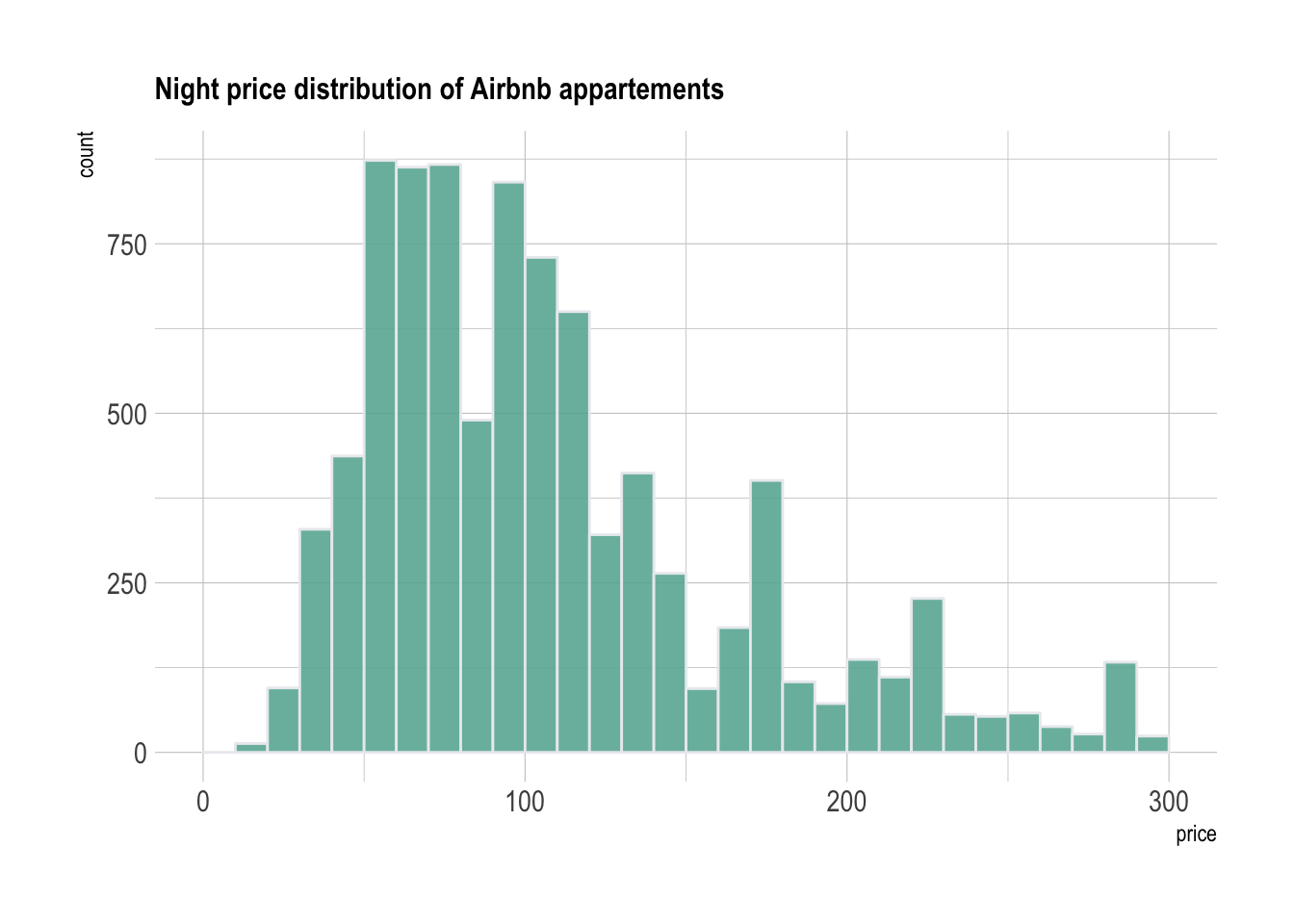A histogram takes as input a numeric variable and cuts it into several bins. The number of observations in each bin is represented by the height of the bar. It is a very common type of graphic and most tools select a bin size value by default.

However, this bin size choice can have a strong impact on the chart insight. Let’s look at the distribution of Airbnb night prices on the French Riviera:

``````# Libraries
library(tidyverse)
library(hrbrthemes)
library(kableExtra)
options(knitr.table.format = "html")

# Load dataset from github

data %>%
filter( price<300 ) %>%
ggplot( aes(x=price)) +
stat_bin(breaks=seq(0,300,10), fill="#69b3a2", color="#e9ecef", alpha=0.9) +
ggtitle("Night price distribution of Airbnb appartements") +
theme_ipsum() +
theme(
plot.title = element_text(size=12)
)``````The price ranges between 10 and 300 euros, with most of the apartments ranging between 60 and 150 euros per night. In this chart, prices are cut in several 10 euro bins: between 0 and 10 euros a night, between 10 and 20, and so on. This is represented on the X-axis. Then, the number of apartments per bin is counted and represented by the Y-axis.

Let’s check what happens when prices are split into bins of 2 euros instead of 10:

``````data %>%
filter( price<300 ) %>%
ggplot( aes(x=price)) +
stat_bin(breaks=seq(0,300,3), fill="#69b3a2", color="#e9ecef", alpha=0.9) +
ggtitle("Night price distribution of Airbnb appartements") +
theme_ipsum() +
theme(
plot.title = element_text(size=12)
)``````